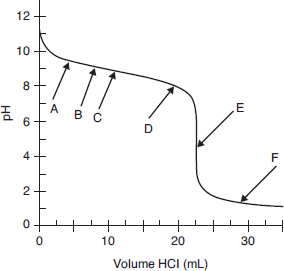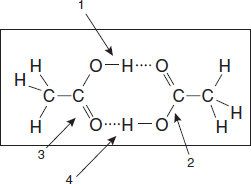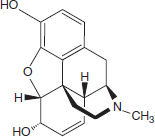# AP Chemistry Practice Test 28

### Test Information10 questions15 minutes

`Questions 1-5 refer to the following information.Questions refer to the titration curve below, which is of a weak base titrated with a strong acid.The pH was measured with a pH meter after small volumes of 0.125 M HCl were added to 25.0 mL of a weak base. Data from that experiment are shown in the above graph.`

1. Which of the following describes the base that is being titrated?

2. Between which two points on the titration curve can the solution be described as a buffer?

3. For the best results with a visual indicator (one that changes color), what will optimize the results?

4. If the student uses a pH indicator that changes color from pH 6 to 8, which statement best characterizes the expected observations?

5. Which arrow points to the place on the curve where pH = pKaof the conjugate acid of the base?

6.In the diagram above, which labeled arrow is pointing toward a covalent bond and which is pointing toward a hydrogen bond?

Covalent Bond   Hydrogen Bond

7. Which would be the easiest way to burn a copper penny?

8. S(s) + O2(g) → SO2(g)

The equation for the reaction between sulfur and oxygen, written in equation form above, can be interpreted in all of the following ways except

9.Morphine, C17H19NO3 (shown above), has a Kb = 8.0 × 10-7. If a 0.00100 M solution of morphine is prepared, the expected pH will be in which one of the following pH ranges?

10. What is the pKa of morphine hydrochloride, C17H20NO3+Cl-, where C17H20NO3+ is the conjugate acid of the morphine in the previous question?